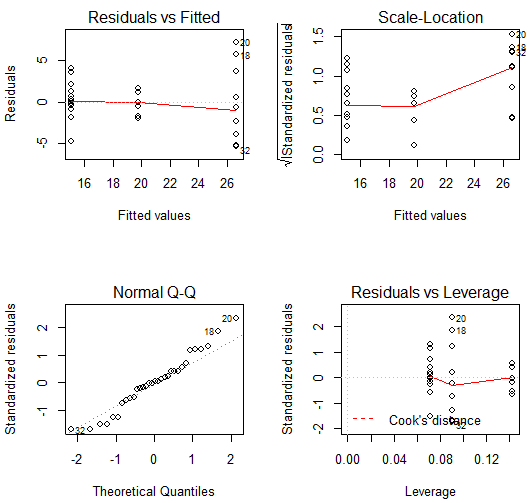# One-Way ANOVA in R

The two-sample t or z test is used to compare two groups from the independent population. However, if there are more than two groups, analysis of variance (ANOVA) can be used.

The statistical test statistic associated with ANOVA is the F-test (also called F-ratio). In Anova procedure an observed F-value is computed and then compared with a critical F-value derived from the relevant F-distribution. The F-value comes from a family of F-distribution defined by two numbers (the degrees of freedom). Note that the F-distribution cannot be negative as it is the ratio of variance and variances are always positive numbers.

The One-Way ANOVA is also known as one-factor ANOVA. It is the extension of independent two samples test for comparing means when there are more than two groups. The data in One-Way ANOVA is organized into several groups base on grouping variable (called factor variable too).

To compute the F-value, the ratio of “the variance between groups”,  and the “variance within groups” need to be computed. The assumptions of Anova should also be checked before performing the ANOVA test. We will learn how to perform One-Way ANOVA in R.

Suppose we are interested in finding the difference of miles per gallon on the bases of numbers of the cylinder in an automobile; from the dataset “mtcars

Let we get some basic insight into the data before performing the ANOVA.

# load and attach the data mtcars
attach(mtcars)
# see the variable names and initial observations
head(mtcars)

Let us find the means of each number of the cylinder group

print(model.tables(res, "means"), digits=4)

Let us draw the boxplot of each group

boxplot(mpg ~ cyl, main="Boxplot", xlab="Number of Cylinders", ylab="mpg")

Now, to perform One-Way ANOVA in R using the aov( ) function. For example,

aov(mpg ~ cyl)

The variable “mpg” is continuous and the variable “cyl” is the grouping variable. From the output note the degrees of freedom under the variable “cyl”. It will be one. It means the results are not correct as the degrees of freedom should be two as there are three groups on “cyl”. In mode (data type) of grouping variable required for ANOVA  should be factor variable. For this purpose, the “cyl” variable can be converted to factor as

cyl <- as.factor(cyl)

Now re-issue the aov( ) function as

aov(mpg ~ cyl)

Now the results will be as required. To get the ANOVA table, use the summary( ) function as

summary(aov (mpg ~ cyl))

Let store the ANOVA results obtained from aov( ) in object say res

res <- aov(mpg ~ cyl)
summary(res)

## Post-Hoc Analysis

Post-hoc tests or multiple-pairwise comparison tests help in finding out which groups differ (significantly) from one other and which do not. The Post-hoc tests allow for multiple-pairwise comparisons without inflating the type-I error. To understand it, suppose the level of significance (type-I error) is 5%. Then the probability of making at least one Type-I error (assuming independence of three events), the maximum family-wise error rate will be

$1-(0.95 \times 0.95 \times 0.95) = 14.2%$

It will give the probability of having at least one FALSE alarm (type-I error).

To perform Tykey’s Post-hoc test and plot the group’s differences in means from Tukey’s test.

# Tukey Honestly Significant Differences
TukeyHSD(res)
plot(TukeyHSD(res))

## Diagnostic Plots

The diagnostic plots can be used to check the assumption of heteroscedasticity, normality, and influential observations.

layout(matrix(c(1,2,3,4), 2,2))
plot(res)## Levene’s Test

To check the assumption of ANOVA, Levene’s test can be used. For this purpose leveneTest( ) function can be used which is available in the car package.

library(car)
leveneTest(res)